## Uniform Circular Motion

If α is the measure of a central angle of a circle, measured in radians, then the length of the intercepted arc (s) can be found by multiplying the radius of the circle (r) by the size of the central angle (α); s = rα. Remember, α must be measured in radians.

Example 1: Find the length (s) of the arc intercepted by a central angle of size 3 radians if the radius of the circle is 5 centimeters (see Figure  1).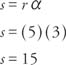Figure  1
Drawing for Example 1

Thus, the length of the intercepted arc is 15 centimeters.

Example 2: Using Figure 2 , find the length (s) of the arc intercepted by a central angle of size − 100° if the radius of the circle is 7 centimeters. Round the answer to two decimal places. (In this problem, the negative value of the angle and the arc length refer to a negative direction.)Figure  2
Drawing for Example 2.

First, convert − 100° to radian measure.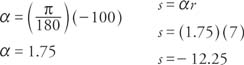Thus, the length of the intercepted arc is −12.25 centimeters.

The linear velocity (v) of a point traveling at a constant speed along an arc of a circle is given as: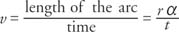Example 3: If the earth has a radius of 4,050 miles and rotates one complete revolution (2π radians) each 24 hours, what is the linear velocity of an object located on the equator?Thus, the linear velocity of the object is 1,060 miles per hour.

The angular velocity (ω) of a point traveling at a constant speed along an arc of a circle is given as: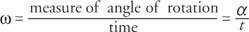Angular and linear velocity are both positive if the movement is counterclockwise and negative if the movement is clockwise.

Example 4: Point P revolves counterclockwise around a point 0 making 7 complete revolutions in 5 seconds. If the radius of the circle shown in Figure 3 is 8 centimeters, find the linear and angular velocities of point P. Approximate π to two decimal places.Figure  3
Drawing for Example 4.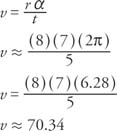Thus, the linear velocity is approximately 70.34 centimeters per second.Thus, the angular velocity is approximately 8.79 radians per second